非常实用，不扯任何理论概念 不包含python基础教程，numpy pandas等常见已经中文化很好的部分知识。

### Import¶

In :
from plotly.offline import download_plotlyjs, init_notebook_mode, plot, iplot
from sklearn.metrics import classification_report
from sklearn.metrics import confusion_matrix
from sklearn.metrics import accuracy_score
import matplotlib.pyplot as plt
import plotly.graph_objs as go
import plotly.plotly as py
import scipy.stats as stats
import seaborn as sns
import pandas as pd
import numpy as np
import matplotlib
import warnings
import sklearn
import scipy
import json
import sys
import csv
import os


### Version¶

In :
print('matplotlib: {}'.format(matplotlib.__version__))
print('sklearn: {}'.format(sklearn.__version__))
print('scipy: {}'.format(scipy.__version__))
print('seaborn: {}'.format(sns.__version__))
print('pandas: {}'.format(pd.__version__))
print('numpy: {}'.format(np.__version__))
print('Python: {}'.format(sys.version))

matplotlib: 3.0.2
sklearn: 0.20.0
scipy: 1.1.0
seaborn: 0.9.0
pandas: 0.23.4
numpy: 1.15.4
Python: 3.7.0 (default, Aug 25 2018, 09:46:22)
[Clang 10.0.0 (clang-1000.10.43.1)]


## Seaborn与Matplotlib¶

Seaborn 是Matplotlib的补充，它专门针对统计数据可视化。但它更进一步：Seaborn扩展了Matplotlib，这就是为什么它可以解决与Matplotlib合作的两个最大挫折。或者，正如迈克尔·瓦斯科姆在“Seaborn的介绍”中所说的那样：“如果matplotlib试图让事情变得容易而且事情变得容易”，那么seaborn也试图制定一套定义明确的硬件。“

Seaborn有助于解决Matplotlib面临的两个主要问题;问题是

Seaborn的重要特征 Seaborn构建于Python的核心可视化库Matplotlib之上。它旨在作为补充，而不是替代。然而，Seaborn带有一些非常重要的功能。我们在这里看一些。这些功能有助于 -

In :
def sinplot(flip = 1):
x = np.linspace(0, 14, 100)
for i in range(1, 5):
plt.plot(x, np.sin(x + i * .5) * (7 - i) * flip)
sinplot()
plt.show()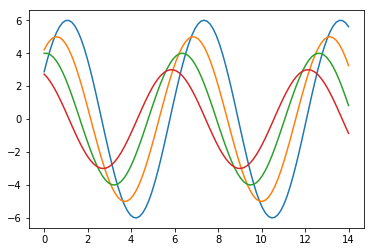In :
def sinplot(flip = 1):
x = np.linspace(0, 14, 100)
for i in range(1, 5):
plt.plot(x, np.sin(x + i * .5) * (7 - i) * flip)

sns.set()
sinplot()
plt.show()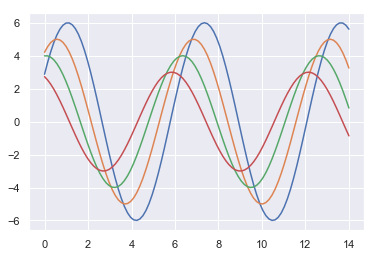In :
np.random.seed(1234)

v1 = pd.Series(np.random.normal(0,10,1000), name='v1')
v2 = pd.Series(2*v1 + np.random.normal(60,15,1000), name='v2')

In :
plt.figure()
plt.hist(v1, alpha=0.7, bins=np.arange(-50,150,5), label='v1');
plt.hist(v2, alpha=0.7, bins=np.arange(-50,150,5), label='v2');
plt.legend();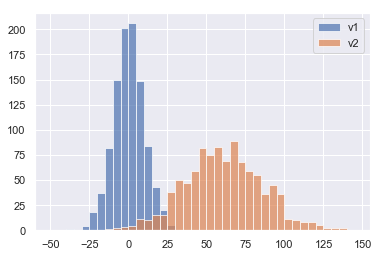In :
plt.figure()
# we can pass keyword arguments for each individual component of the plot
sns.distplot(v2, hist_kws={'color': 'Teal'}, kde_kws={'color': 'Navy'});

/usr/local/Cellar/python/3.7.0/Frameworks/Python.framework/Versions/3.7/lib/python3.7/site-packages/scipy/stats/stats.py:1713: FutureWarning:

Using a non-tuple sequence for multidimensional indexing is deprecated; use arr[tuple(seq)] instead of arr[seq]. In the future this will be interpreted as an array index, arr[np.array(seq)], which will result either in an error or a different result.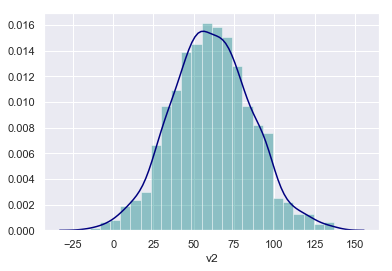In :
sns.jointplot(v1, v2, alpha=0.4);

/usr/local/Cellar/python/3.7.0/Frameworks/Python.framework/Versions/3.7/lib/python3.7/site-packages/scipy/stats/stats.py:1713: FutureWarning:

Using a non-tuple sequence for multidimensional indexing is deprecated; use arr[tuple(seq)] instead of arr[seq]. In the future this will be interpreted as an array index, arr[np.array(seq)], which will result either in an error or a different result.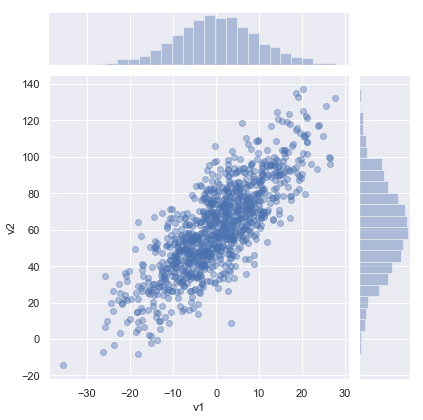In :
grid = sns.jointplot(v1, v2, alpha=0.4);
grid.ax_joint.set_aspect('equal')

/usr/local/Cellar/python/3.7.0/Frameworks/Python.framework/Versions/3.7/lib/python3.7/site-packages/scipy/stats/stats.py:1713: FutureWarning:

Using a non-tuple sequence for multidimensional indexing is deprecated; use arr[tuple(seq)] instead of arr[seq]. In the future this will be interpreted as an array index, arr[np.array(seq)], which will result either in an error or a different result.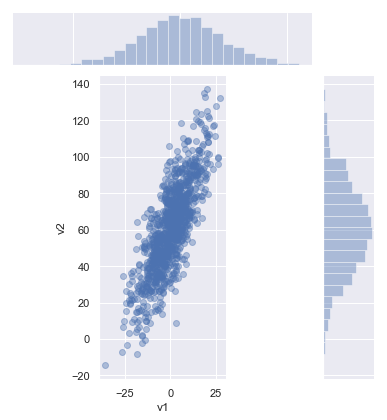In :
sns.jointplot(v1, v2, kind='hex');

/usr/local/Cellar/python/3.7.0/Frameworks/Python.framework/Versions/3.7/lib/python3.7/site-packages/scipy/stats/stats.py:1713: FutureWarning:

Using a non-tuple sequence for multidimensional indexing is deprecated; use arr[tuple(seq)] instead of arr[seq]. In the future this will be interpreted as an array index, arr[np.array(seq)], which will result either in an error or a different result.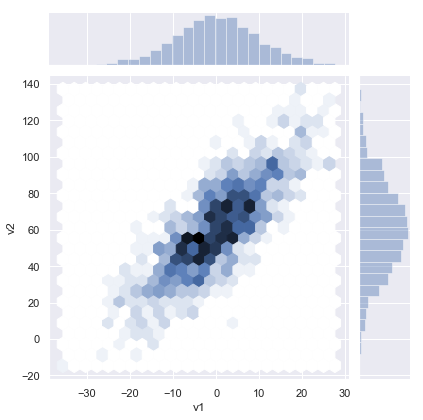In :
# set the seaborn style for all the following plots
sns.set_style('white')

sns.jointplot(v1, v2, kind='kde', space=0);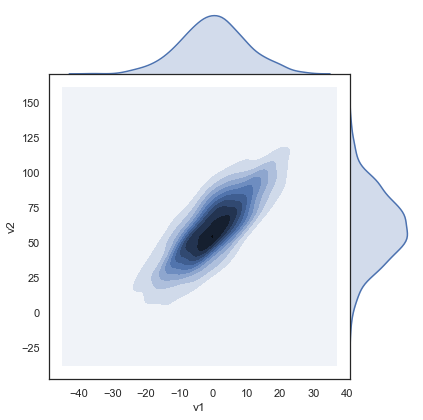In :
train = pd.read_csv('../input/train.csv')
train.head()

Out:
PassengerId Survived Pclass Name Sex Age SibSp Parch Ticket Fare Cabin Embarked
0 1 0 3 Braund, Mr. Owen Harris male 22.0 1 0 A/5 21171 7.2500 NaN S
1 2 1 1 Cumings, Mrs. John Bradley (Florence Briggs Th... female 38.0 1 0 PC 17599 71.2833 C85 C
2 3 1 3 Heikkinen, Miss. Laina female 26.0 0 0 STON/O2. 3101282 7.9250 NaN S
3 4 1 1 Futrelle, Mrs. Jacques Heath (Lily May Peel) female 35.0 1 0 113803 53.1000 C123 S
4 5 0 3 Allen, Mr. William Henry male 35.0 0 0 373450 8.0500 NaN S

## SKlearn¶

• 开源 Python生态系统提供了一个独立，多功能和强大的科学工作环境，包括：[NumPy]，[SciPy]，[IPython]，[Matplotlib]，[Pandas]

### 简介¶

• Scikit-Learn构建于NumPy和SciPy之上，通过机器学习算法补充这一科学环境;
• 根据设计，Scikit-Learn 非侵入式，易于使用且易于与其他库结合使用;
• 核心算法以低级语言实现。

### 算法¶

......还有更多！（参见我们的[参考资料]（http://scikit-learn.org/dev/modules/classes.html））

### 框架¶

$$Err(\varphi) = \mathbb{E}_{X,Y}\{ \ell(Y, \varphi(X)) \}$$

### 需求¶

• 对背景事件的信号进行分类;
• 从症状诊断疾病;
• 识别照片中的猫;
• 使用Kinect摄像头识别身体部位;
• ......

### 数据¶

• 输入数据= Numpy数组或Scipy稀疏矩阵;
• 使用矩阵或向量上定义的高级操作表示算法（类似于MATLAB）;      - 利用高效的低杠杆实施;      - 保持代码简短易读。
In :
from sklearn import datasets
iris = datasets.load_iris()
X_iris = iris.data
y_iris = iris.target


In :
print (X_iris.shape, y_iris.shape)
print ('Feature names:{0}'.format(iris.feature_names))
print ('Target classes:{0}'.format(iris.target_names))
print ('First instance features:{0}'.format(X_iris))

(150, 4) (150,)
Feature names:['sepal length (cm)', 'sepal width (cm)', 'petal length (cm)', 'petal width (cm)']
Target classes:['setosa' 'versicolor' 'virginica']
First instance features:[5.1 3.5 1.4 0.2]


In :
plt.figure('sepal')
colormarkers = [ ['red','s'], ['greenyellow','o'], ['blue','x']]
for i in range(len(colormarkers)):
px = X_iris[:, 0][y_iris == i]
py = X_iris[:, 1][y_iris == i]
plt.scatter(px, py, c=colormarkers[i], marker=colormarkers[i])

plt.title('Iris Dataset: Sepal width vs sepal length')
plt.legend(iris.target_names)
plt.xlabel('Sepal length')
plt.ylabel('Sepal width')
plt.figure('petal')

for i in range(len(colormarkers)):
px = X_iris[:, 2][y_iris == i]
py = X_iris[:, 3][y_iris == i]
plt.scatter(px, py, c=colormarkers[i], marker=colormarkers[i])

plt.title('Iris Dataset: petal width vs petal length')
plt.legend(iris.target_names)
plt.xlabel('Petal length')
plt.ylabel('Petal width')
plt.show()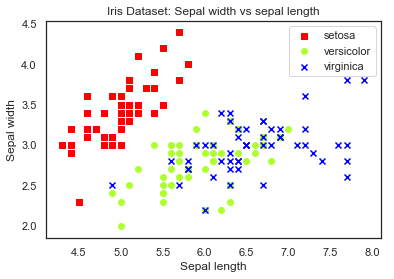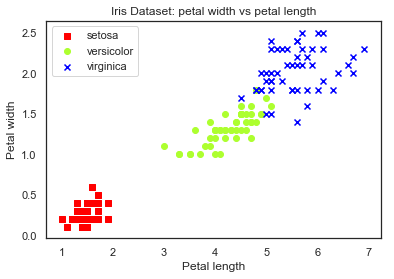### 监督学习：分类¶

1936年，罗纳德·费希尔爵士将统计数据集引入统计领域，用它来开发线性判别模型。他所做的是建立一个将物种与其他物种分开的属性的线性组合，即找到一条类似于我们在上一节中建议的直线。

• 选择你的属性，
• 根据可用数据构建模型，和
• 评估您的模型在以前看不见的数据上的表现。

### 单独的培训和测试集¶

In :
from sklearn.model_selection import train_test_split
from sklearn import preprocessing

# Create dataset with only the first two attributes
X, y = X_iris[:, [0,1]], y_iris
# Test set will be the 25% taken randomly
X_train, X_test, y_train, y_test = train_test_split(X, y, test_size=0.25, random_state=33)

# Standarize the features
scaler = preprocessing.StandardScaler().fit(X_train)
X_train = scaler.transform(X_train)
X_test = scaler.transform(X_test)


In :
print ('Training set mean:{:.2f} and standard deviation:{:.2f}'.format(np.average(X_train),np.std(X_train)))
print ('Testing set mean:{:.2f} and standard deviation:{:.2f}'.format(np.average(X_test),np.std(X_test)))

Training set mean:0.00 and standard deviation:1.00
Testing set mean:0.13 and standard deviation:0.71


In :
colormarkers = [ ['red','s'], ['greenyellow','o'], ['blue','x']]
plt.figure('Training Data')
for i in range(len(colormarkers)):
xs = X_train[:, 0][y_train == i]
ys = X_train[:, 1][y_train == i]
plt.scatter(xs, ys, c=colormarkers[i], marker=colormarkers[i])

plt.title('Training instances, after scaling')
plt.legend(iris.target_names)
plt.xlabel('Sepal length')
plt.ylabel('Sepal width')
plt.show()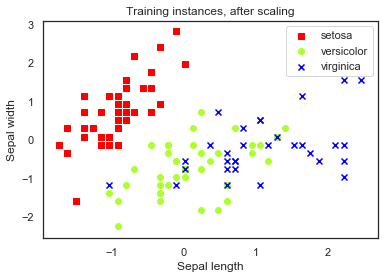### 线性二元分类器¶

In :
import copy
y_train_setosa = copy.copy(y_train)
# Every 1 and 2 classes in the training set will became just 1
y_train_setosa[y_train_setosa > 0]=1
y_test_setosa = copy.copy(y_test)
y_test_setosa[y_test_setosa > 0]=1

print ('New training target classes:\n{0}'.format(y_train_setosa))

New training target classes:
[1 0 1 1 1 0 0 1 0 1 0 0 1 1 0 1 1 1 1 1 0 0 1 0 0 1 1 1 1 1 1 1 0 0 1 1 0
1 1 1 1 0 1 0 1 0 1 1 0 1 1 0 0 1 0 0 0 1 1 0 1 0 1 0 1 1 1 1 1 0 1 0 1 1
0 0 0 0 1 1 0 1 1 1 1 0 0 1 1 1 0 1 1 0 1 1 1 1 1 0 1 0 0 0 1 1 1 1 1 1 1
0]


1847年，路易斯·奥古斯丁·柯西（Louis Augustin Cauchy）引入了“梯度下降”（Gradient Descent）来求解线性方程组。这个想法是基于观察到多变量函数在其负梯度方向上减小最快（您可以将梯度视为几个维度的导数的推广）。如果我们想要找到它的最小值（至少是局部值），我们可以向其负梯度方向移动。这正是梯度下降所做的。

scikit-learn中的每个分类器都以相同的方式创建：使用分类器的可配置超参数调用方法来创建分类器的实例。在这种情况下，我们将使用linear _model.SGDClassifier，告诉scikit-learn使用 log loss函数。

In :
from sklearn import linear_model
clf = linear_model.SGDClassifier(loss='log', random_state=42)
print (clf)

SGDClassifier(alpha=0.0001, average=False, class_weight=None,
early_stopping=False, epsilon=0.1, eta0=0.0, fit_intercept=True,
l1_ratio=0.15, learning_rate='optimal', loss='log', max_iter=None,
n_iter=None, n_iter_no_change=5, n_jobs=None, penalty='l2',
power_t=0.5, random_state=42, shuffle=True, tol=None,
validation_fraction=0.1, verbose=0, warm_start=False)


In :
clf.fit(X_train, y_train_setosa)


/usr/local/Cellar/python/3.7.0/Frameworks/Python.framework/Versions/3.7/lib/python3.7/site-packages/sklearn/linear_model/stochastic_gradient.py:144: FutureWarning:

max_iter and tol parameters have been added in SGDClassifier in 0.19. If both are left unset, they default to max_iter=5 and tol=None. If tol is not None, max_iter defaults to max_iter=1000. From 0.21, default max_iter will be 1000, and default tol will be 1e-3.


Out:
SGDClassifier(alpha=0.0001, average=False, class_weight=None,
early_stopping=False, epsilon=0.1, eta0=0.0, fit_intercept=True,
l1_ratio=0.15, learning_rate='optimal', loss='log', max_iter=None,
n_iter=None, n_iter_no_change=5, n_jobs=None, penalty='l2',
power_t=0.5, random_state=42, shuffle=True, tol=None,
validation_fraction=0.1, verbose=0, warm_start=False)

In :
print (clf.coef_,clf.intercept_)

[[ 31.0790909  -17.78632765]] [17.31337552]


### 预测¶

In :
print ('If the flower has 4.7 petal width and 3.1 petal length is a {}'.format(
iris.target_names[clf.predict(scaler.transform([[4.7, 3.1]]))]))

If the flower has 4.7 petal width and 3.1 petal length is a ['setosa']


### 回到原来的三级问题¶

In :
clf2 = linear_model.SGDClassifier(loss='log', random_state=33)
clf2.fit(X_train, y_train)
print (len(clf2.coef_))

3

/usr/local/Cellar/python/3.7.0/Frameworks/Python.framework/Versions/3.7/lib/python3.7/site-packages/sklearn/linear_model/stochastic_gradient.py:144: FutureWarning:

max_iter and tol parameters have been added in SGDClassifier in 0.19. If both are left unset, they default to max_iter=5 and tol=None. If tol is not None, max_iter defaults to max_iter=1000. From 0.21, default max_iter will be 1000, and default tol will be 1e-3.



Let us evaluate on the previous instance to find the three-class prediction. Scikit-learn tries the three classifiers.

In :
scaler.transform([[4.7, 3.1]])
print(clf2.decision_function(scaler.transform([[4.7, 3.1]])))
clf2.predict(scaler.transform([[4.7, 3.1]]))

[[ 13.56767487   1.74380721 -37.36375592]]

Out:
array()

decision_function方法告诉我们分类器得分（在我们的例子中，决策边界不等式的左侧）。在我们的例子中，第一个分类器说花是一个setosa（我们有一个大于零的分数），它不是一个versicolor也不是virginica。简单。如果我们有两个积极的价值怎么办？在我们的例子中，最高分将是远离决策线的点。

### 评估分类器¶

In :
from sklearn import metrics
y_train_pred = clf2.predict(X_train)
print ('Accuracy on the training set:{:.2f}'.format(metrics.accuracy_score(y_train, y_train_pred)))

Accuracy on the training set:0.82


In :
y_pred = clf2.predict(X_test)
print ('Accuracy on the training set:{:.2f}'.format(metrics.accuracy_score(y_test, y_pred)))

Accuracy on the training set:0.68


In :
print (metrics.confusion_matrix(y_test, y_pred))

[[ 8  0  0]
[ 0  3  8]
[ 0  4 15]]


In :
print (metrics.classification_report(y_test, y_pred, target_names=iris.target_names))

              precision    recall  f1-score   support

setosa       1.00      1.00      1.00         8
versicolor       0.43      0.27      0.33        11
virginica       0.65      0.79      0.71        19

micro avg       0.68      0.68      0.68        38
macro avg       0.69      0.69      0.68        38
weighted avg       0.66      0.68      0.66        38


• 精确计算预测为正确评估的正数的实例的比例（它衡量我们的分类器在表示实例为正时的权利）。
• 召回计算正确评估的正面实例的比例（测量分类器在面对正面实例时的正确性）。
• F1分数是精度和召回的调和平均值，并尝试将两者合并为一个数字。

### 使用四个花属性¶

In :
# Test set will be the 25% taken randomly
X_train4, X_test4, y_train4, y_test4 = train_test_split(X_iris, y_iris, test_size=0.25, random_state=33)

# Standarize the features
scaler = preprocessing.StandardScaler().fit(X_train4)
X_train4 = scaler.transform(X_train4)
X_test4 = scaler.transform(X_test4)

# Build the classifier
clf3 = linear_model.SGDClassifier(loss='log', random_state=33)
clf3.fit(X_train4, y_train4)

# Evaluate the classifier on the evaluation set
y_pred4 = clf3.predict(X_test4)
print (metrics.classification_report(y_test4, y_pred4, target_names=iris.target_names))

              precision    recall  f1-score   support

setosa       1.00      1.00      1.00         8
versicolor       0.78      0.64      0.70        11
virginica       0.81      0.89      0.85        19

micro avg       0.84      0.84      0.84        38
macro avg       0.86      0.84      0.85        38
weighted avg       0.84      0.84      0.84        38


/usr/local/Cellar/python/3.7.0/Frameworks/Python.framework/Versions/3.7/lib/python3.7/site-packages/sklearn/linear_model/stochastic_gradient.py:144: FutureWarning:

max_iter and tol parameters have been added in SGDClassifier in 0.19. If both are left unset, they default to max_iter=5 and tol=None. If tol is not None, max_iter defaults to max_iter=1000. From 0.21, default max_iter will be 1000, and default tol will be 1e-3.



### 无监督学习：聚类¶

K-means可能是最流行的聚类算法，因为它非常简单易于实现，并且在不同的任务中表现出良好的性能。它属于分区算法类，它同时将数据点分成不同的组，称为集群。我们将k-means应用于训练数据，仅使用sepal维度，构建3个集群（请注意，我们可以选择不同数量的集群来将数据分组到）

In :
from sklearn import cluster
clf_sepal = cluster.KMeans(init='k-means++', n_clusters=3, random_state=33)
clf_sepal.fit(X_train4[:,0:2])

Out:
KMeans(algorithm='auto', copy_x=True, init='k-means++', max_iter=300,
n_clusters=3, n_init=10, n_jobs=None, precompute_distances='auto',
random_state=33, tol=0.0001, verbose=0)

In :
print (clf_sepal.labels_)

[1 0 1 1 1 0 0 1 0 2 0 0 1 2 0 2 1 2 1 0 0 1 1 0 0 2 0 1 2 2 1 1 0 0 2 1 0
1 1 2 1 0 2 0 1 0 2 2 0 2 1 0 0 1 0 0 0 2 1 0 1 0 1 0 1 2 1 1 1 0 1 0 2 1
0 0 0 0 2 2 0 1 1 2 1 0 0 1 1 1 0 1 1 0 2 1 2 1 2 0 2 0 0 0 1 1 2 1 1 1 2
0]


In :
print (y_train4[clf_sepal.labels_==0])

[0 0 0 0 0 0 0 1 0 0 0 1 0 0 0 0 0 0 0 0 0 0 0 0 0 0 0 0 0 0 0 0 0 0 0 0 0
0 0 0 0 0 0]

In :
print (y_train4[clf_sepal.labels_==1])

[1 1 1 1 1 1 2 1 0 2 1 2 2 1 1 2 2 1 2 2 2 1 1 1 1 1 1 1 2 1 1 1 1 1 1 1 1
2 1 2 1 1 2 1]

In :
print (y_train4[clf_sepal.labels_==2])

[2 2 1 2 2 2 2 1 1 2 2 1 2 2 1 1 2 2 2 2 2 2 1 2 2]


In :
colormarkers = [ ['red','s'], ['greenyellow','o'], ['blue','x']]
step = .01
margin = .1
sl_min, sl_max = X_train4[:, 0].min()-margin, X_train4[:, 0].max() + margin
sw_min, sw_max = X_train4[:, 1].min()-margin, X_train4[:, 1].max() + margin
sl, sw  = np.meshgrid(
np.arange(sl_min, sl_max, step),
np.arange(sw_min, sw_max, step)
)
Zs = clf_sepal.predict(np.c_[sl.ravel(), sw.ravel()]).reshape(sl.shape)
centroids_s = clf_sepal.cluster_centers_


In :
plt.figure(1)
plt.clf()
plt.imshow(Zs, interpolation='nearest', extent=(sl.min(), sl.max(), sw.min(), sw.max()), cmap= plt.cm.Pastel1, aspect='auto', origin='lower')
for j in [0,1,2]:
px = X_train4[:, 0][y_train == j]
py = X_train4[:, 1][y_train == j]
plt.scatter(px, py, c=colormarkers[j], marker= colormarkers[j])
plt.scatter(centroids_s[:, 0], centroids_s[:, 1],marker='*',linewidths=3, color='black', zorder=10)
plt.title('K-means clustering on the Iris dataset using Sepal dimensions\nCentroids are marked with stars')
plt.xlim(sl_min, sl_max)
plt.ylim(sw_min, sw_max)
plt.xlabel("Sepal length")
plt.ylabel("Sepal width")
plt.show()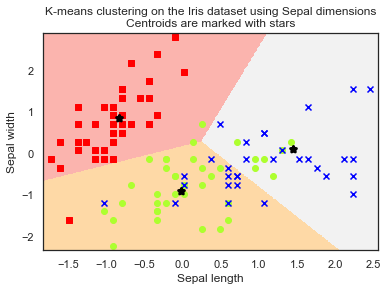In :
clf_petal = cluster.KMeans(init='k-means++', n_clusters=3, random_state=33)
clf_petal.fit(X_train4[:,2:4])

Out:
KMeans(algorithm='auto', copy_x=True, init='k-means++', max_iter=300,
n_clusters=3, n_init=10, n_jobs=None, precompute_distances='auto',
random_state=33, tol=0.0001, verbose=0)
In :
print (y_train4[clf_petal.labels_==0])

[0 0 0 0 0 0 0 0 0 0 0 0 0 0 0 0 0 0 0 0 0 0 0 0 0 0 0 0 0 0 0 0 0 0 0 0 0
0 0 0 0 0]

In :
print (y_train4[clf_petal.labels_==1])

[1 1 1 1 1 1 1 1 2 1 1 1 1 1 1 1 1 1 1 1 1 1 1 1 1 1 1 1 1 1 1 1 1 2 1 1 1
1]

In :
print (y_train4[clf_petal.labels_==2])

[2 2 2 2 1 2 2 2 2 2 2 2 2 2 2 2 2 2 2 2 2 2 2 1 2 2 2 1 2 2 2 2]


In :
colormarkers = [ ['red','s'], ['greenyellow','o'], ['blue','x']]
step = .01
margin = .1
sl_min, sl_max = X_train4[:, 2].min()-margin, X_train4[:, 2].max() + margin
sw_min, sw_max = X_train4[:, 3].min()-margin, X_train4[:, 3].max() + margin
sl, sw  = np.meshgrid(
np.arange(sl_min, sl_max, step),
np.arange(sw_min, sw_max, step),
)
Zs = clf_petal.predict(np.c_[sl.ravel(), sw.ravel()]).reshape(sl.shape)
centroids_s = clf_petal.cluster_centers_
plt.figure(1)
plt.clf()
plt.imshow(Zs, interpolation='nearest', extent=(sl.min(), sl.max(), sw.min(), sw.max()), cmap= plt.cm.Pastel1, aspect='auto', origin='lower')
for j in [0,1,2]:
px = X_train4[:, 2][y_train4 == j]
py = X_train4[:, 3][y_train4 == j]
plt.scatter(px, py, c=colormarkers[j], marker= colormarkers[j])
plt.scatter(centroids_s[:, 0], centroids_s[:, 1],marker='*',linewidths=3, color='black', zorder=10)
plt.title('K-means clustering on the Iris dataset using Petal dimensions\nCentroids are marked with stars')
plt.xlim(sl_min, sl_max)
plt.ylim(sw_min, sw_max)
plt.xlabel("Petal length")
plt.ylabel("Petal width")
plt.show()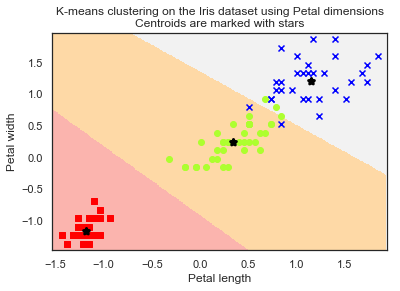In :
clf = cluster.KMeans(init='k-means++', n_clusters=3, random_state=33)
clf.fit(X_train4)

Out:
KMeans(algorithm='auto', copy_x=True, init='k-means++', max_iter=300,
n_clusters=3, n_init=10, n_jobs=None, precompute_distances='auto',
random_state=33, tol=0.0001, verbose=0)
In :
print (y_train[clf.labels_==0])

[0 0 0 0 0 0 0 0 0 0 0 0 0 0 0 0 0 0 0 0 0 0 0 0 0 0 0 0 0 0 0 0 0 0 0 0 0
0 0 0 0 0]

In :
print (y_train[clf.labels_==1])

[1 1 1 1 1 1 1 2 1 2 1 1 1 2 1 1 1 1 1 1 1 1 1 1 1 1 1 1 1 2 1 2 1 1 2 1]

In :
print (y_train[clf.labels_==2])

[2 2 1 2 2 1 2 1 2 2 2 1 2 1 2 2 2 1 2 2 2 2 1 1 2 2 2 2 2 2 2 1 2 2]


In :
y_pred=clf.predict(X_test4)
print (metrics.classification_report(y_test, y_pred, target_names=['setosa','versicolor','virginica']))

              precision    recall  f1-score   support

setosa       1.00      1.00      1.00         8
versicolor       0.58      0.64      0.61        11
virginica       0.78      0.74      0.76        19

micro avg       0.76      0.76      0.76        38
macro avg       0.79      0.79      0.79        38
weighted avg       0.77      0.76      0.77        38


In :
y_pred_petal=clf_petal.predict(X_test4[:,2:4])
print (metrics.classification_report(y_test, y_pred_petal, target_names=['setosa','versicolor','virginica']))

              precision    recall  f1-score   support

setosa       1.00      1.00      1.00         8
versicolor       0.85      1.00      0.92        11
virginica       1.00      0.89      0.94        19

micro avg       0.95      0.95      0.95        38
macro avg       0.95      0.96      0.95        38
weighted avg       0.96      0.95      0.95        38



### 监督学习：回归¶

In :
from sklearn.datasets import load_boston
boston = load_boston()
print ('Boston dataset shape:{}'.format(boston.data.shape))

Boston dataset shape:(506, 13)

In :
print (boston.feature_names)

['CRIM' 'ZN' 'INDUS' 'CHAS' 'NOX' 'RM' 'AGE' 'DIS' 'RAD' 'TAX' 'PTRATIO'
'B' 'LSTAT']